# 978-0134292380 Chapter 8

Document Type
Test Prep
Book Title
Fundamentals of Hydraulic Engineering Systems 5th Edition
Authors
A. Osman H. Akan, Ned H. C. Hwang, Robert J. Houghtalen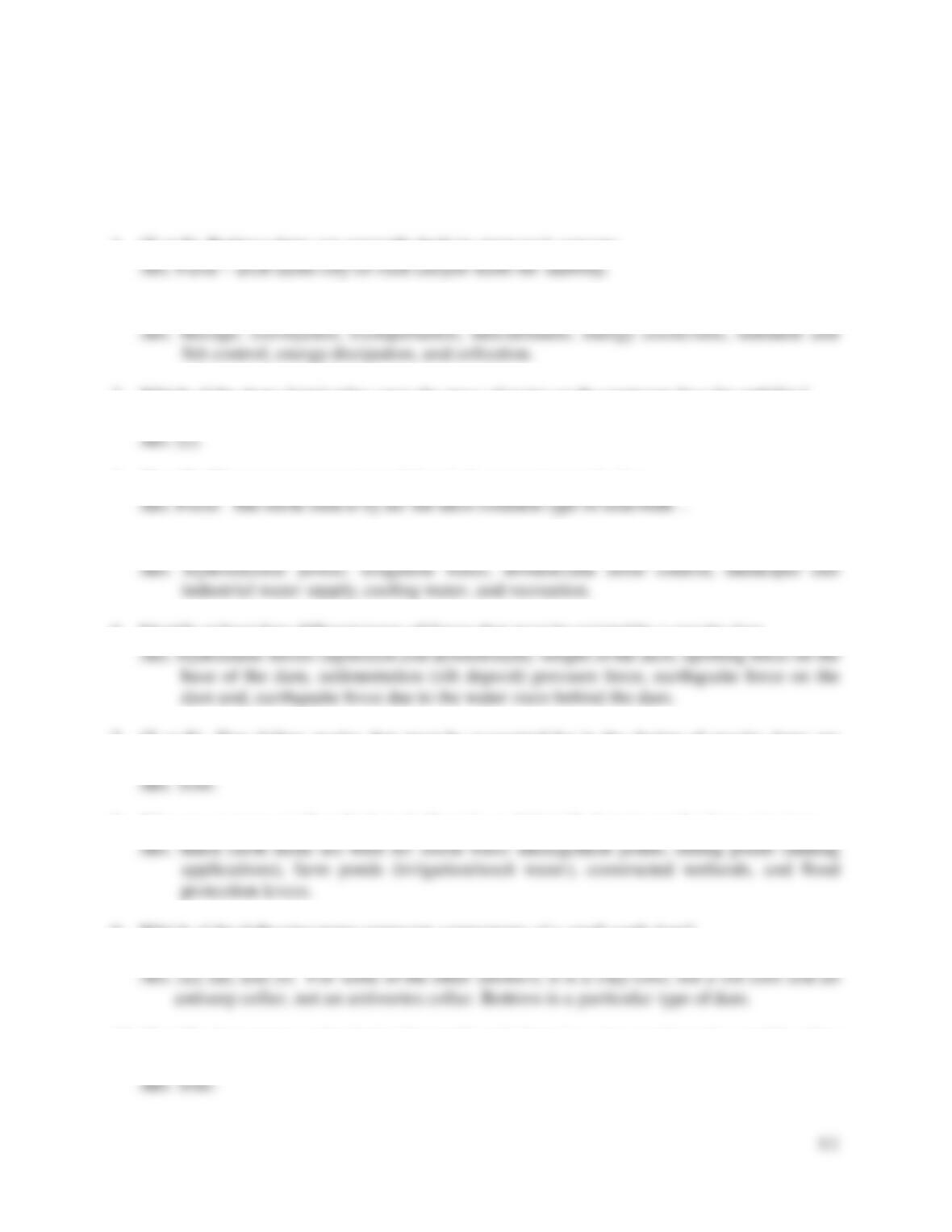80
TEST QUESTIONS & PROBLEMS - CHAPTER #8
1. (T or F) Buttress dams are generally built in steep rock canyons.
2. Identify at least four functions of some common hydraulic structures.
3. Which of the dams listed relies upon the mass of water on the upstream face for stability?
a) gravity b) arch c) buttress
4. (T or F) The most common type of dam is the concrete gravity dam.
5. Identify at least four functions of dams.
6. Identify at least four different types of forces that must be resisted by a gravity dam.
7. (T or F) Two failure modes that must be accounted for in the design of gravity dams are
overturning and sliding.
8. Why are so many small earth dams built each year? Identify 3 purposes for these structures.
9. Which of the following terms represent components of a small earth dam?
a) riser and barrel b) silt core c) antivortex collar d) spillway e) buttress f) key/keyway
10. (T or F) A common outlet device for small earth dams is a riser and barrel assembly, often
called a drop inlet.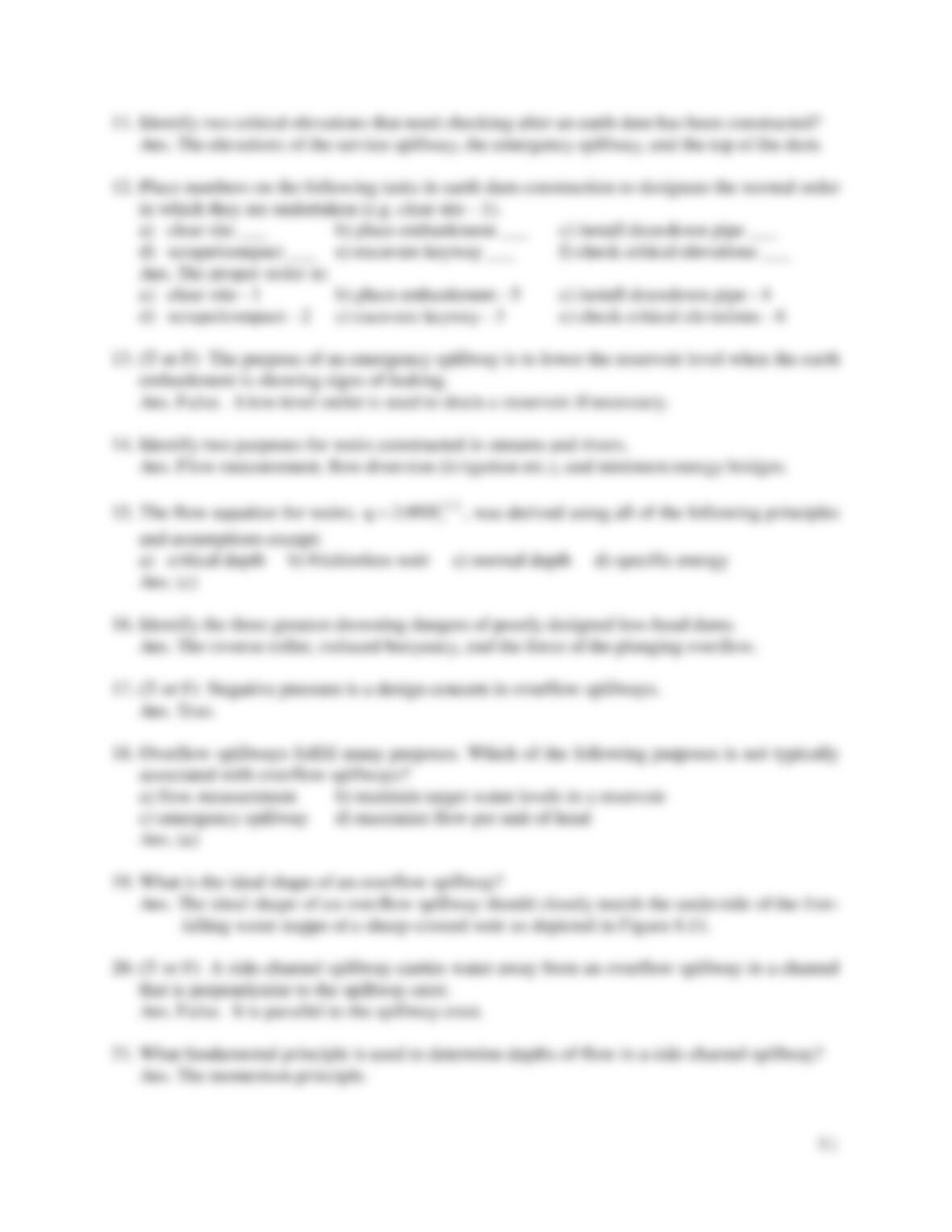11. Identify two critical elevations that need checking after an earth dam has been constructed?
12. Place numbers on the following tasks in earth dam construction to designate the normal order
in which they are undertaken (e.g. clear site - 1).
a) clear site ___ b) place embankment ___ c) install drawdown pipe ___
d) scrape/compact ___ e) excavate keyway ___ f) check critical elevations ___
13. (T or F) The purpose of an emergency spillway is to lower the reservoir level when the earth
embankment is showing signs of leaking.
14. Identify two purposes for weirs constructed in streams and rivers.
15. The flow equation for weirs;
2/3
09.3 s
Hq
, was derived using all of the following principles
and assumptions except:
a) critical depth b) frictionless weir c) normal depth d) specific energy
16. Identify the three greatest drowning dangers of poorly designed low-head dams.
17. (T or F) Negative pressure is a design concern in overflow spillways.
18. Overflow spillways fulfill many purposes. Which of the following purposes is not typically
associated with overflow spillways?
a) flow measurement b) maintain target water levels in a reservoir
c) emergency spillway d) maximize flow per unit of head
19. What is the ideal shape of an overflow spillway?
20. (T or F) A side-channel spillway carries water away from an overflow spillway in a channel
that is perpendicular to the spillway crest.
21. What fundamental principle is used to determine depths of flow in a side-channel spillway?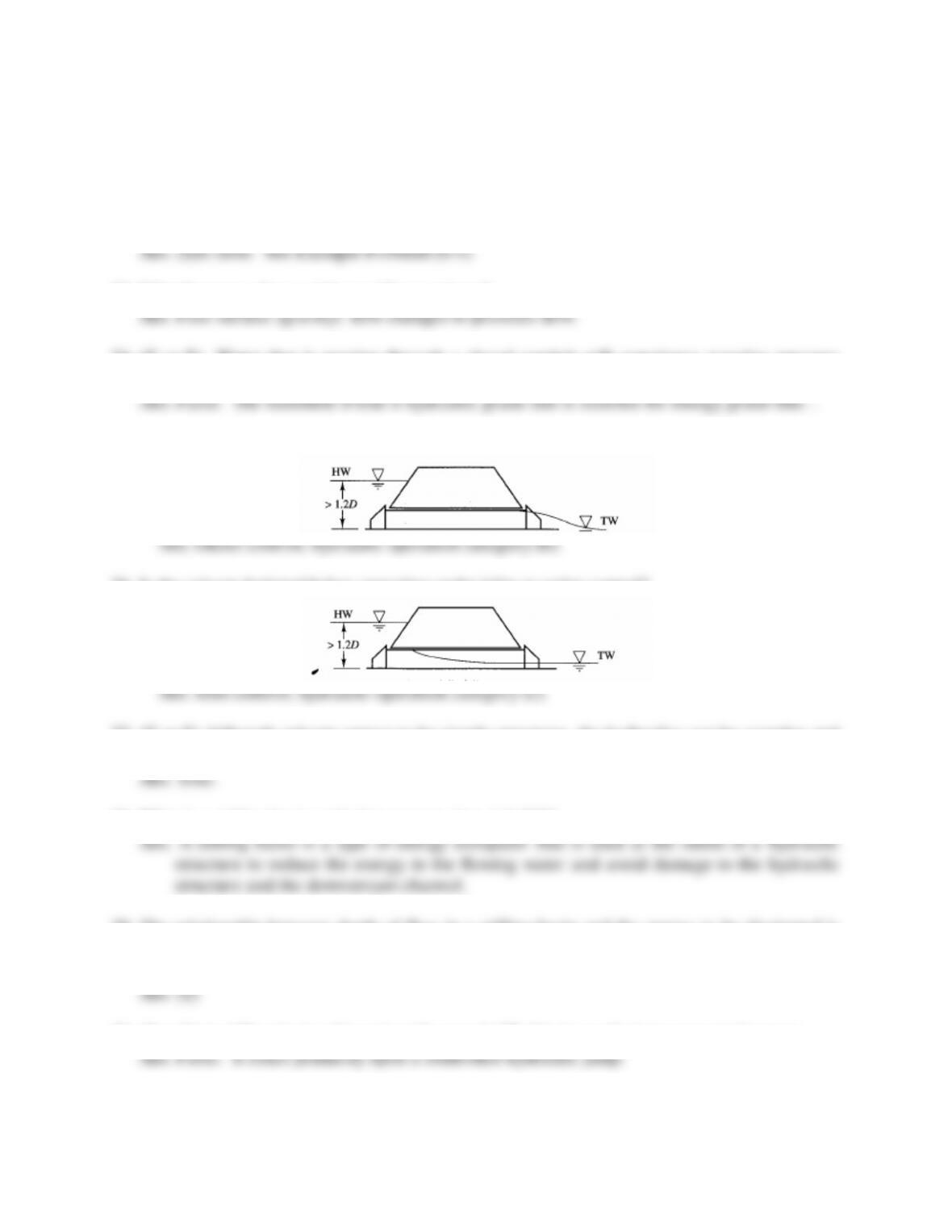82
22. Which of the following statements is not true about siphon spillways when they are
functioning as true siphons?
a) Siphon spillways function like pipes under pressure flow.
b) The head on a siphon spillway does not depend solely on the upstream reservoir level.
c) The crest section of siphon spillways can encounter sub-atmospheric pressure.
d) Siphon spillways require a circular cross sectional flow area, like a pipe.
23. What happens when a siphon spillway primes?
24. (T or F) Water that is passing through a closed conduit will experience negative pressure
when the conduit is elevated above the energy grade line.
25. Is the culvert depicted below operating under inlet or outlet control?
26. Is the culvert depicted below operating under inlet or outlet control?
27. (T or F) Although culverts appear to be simple structures, the hydraulics can be complex and
involve the principles of pressure pipe flow, orifice flow, and open channel flow.
28. What is a stilling basin and what purpose does it fulfill?
29. The relationship between depth of flow in a stilling basin and the energy to be dissipated is
contained in which of the flowing relationships?
a) Froude number b) critical depth c) normal depth d) specific energy
30. (T or F) A stilling basin relies primarily upon baffle blocks to dissipate unwanted energy.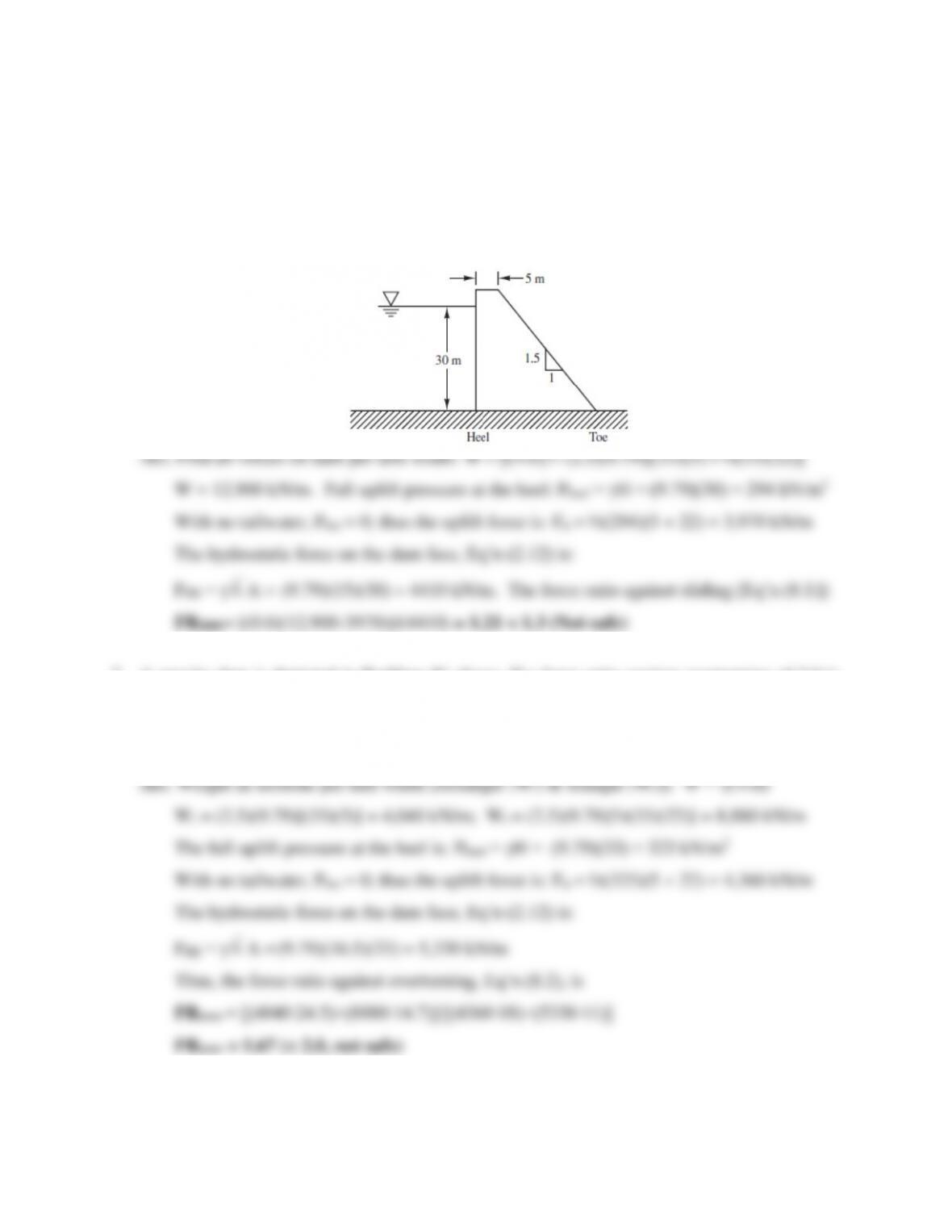83
Problems
1. A gravity dam is depicted in the figure below. If a force ratio against sliding of 1.3 is
required, determine whether the 33 m high dam is safe. Assume the coefficient of friction
between the dam base and the foundation is 0.6, the specific gravity of concrete is 2.5, and
full uplift forces exist on the base of the dam. Neglect earthquake and sedimentation forces.
2. A gravity dam is depicted in Problem #1 above. If a force ratio against overturning of 2.0 is
required, determine whether the dam is safe if the water level rises to the top of the 33 m high
dam during a flood event. Assume the specific gravity of concrete is 2.5, and full uplift
forces exist on the base of the dam. Neglect earthquake and sedimentation forces.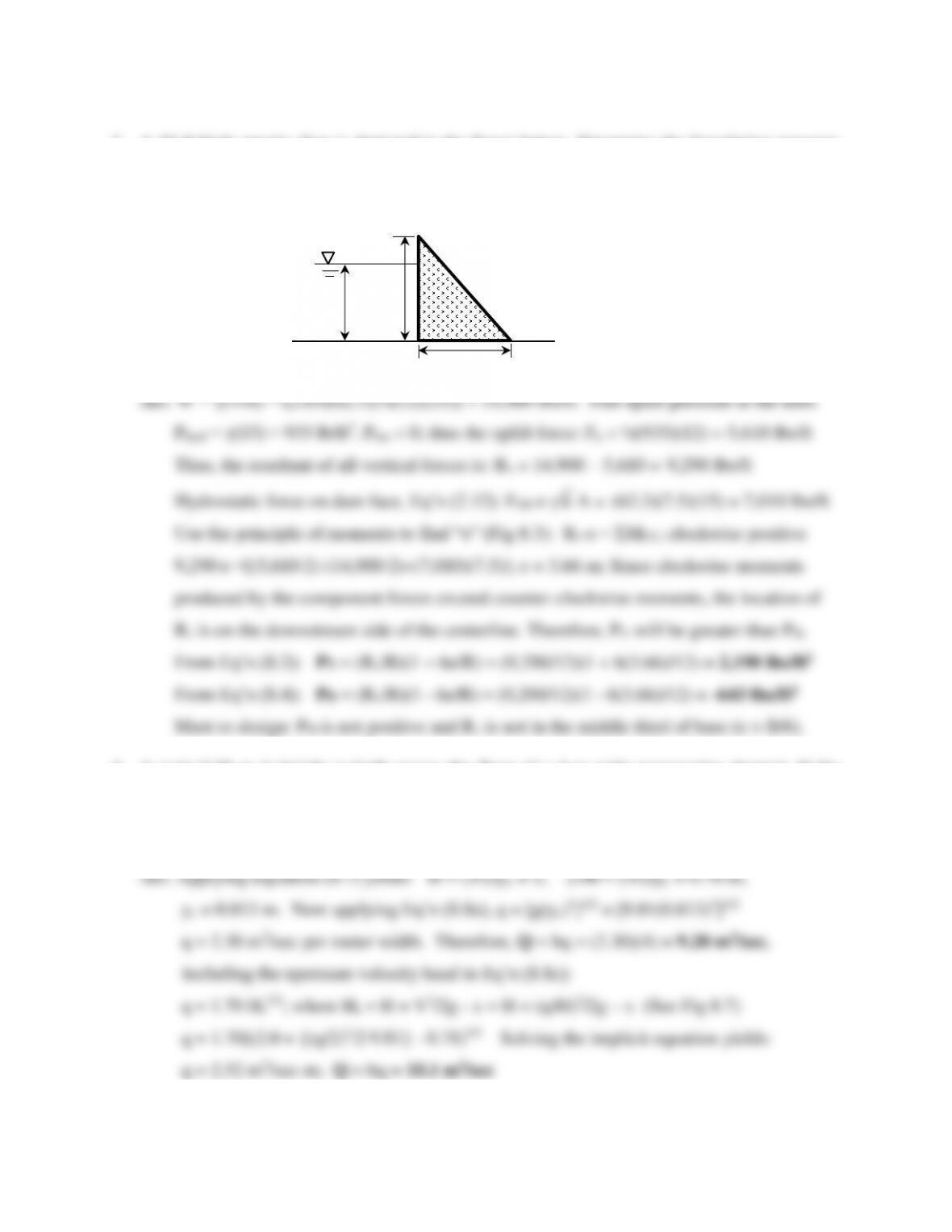84
3. A 15-ft-high gravity dam is depicted in the figure below. Determine the foundation pressure
at the heel and toe of the dam if the water behind the dam rises to a depth of 15 ft. Assume
the coefficient of friction between the 12-ft-long dam base and the foundation is 0.6, the
specific gravity of concrete is 2.65, and full uplift forces exist on the base of the dam.
4. A weir 0.78 m in height is built across the floor of a 4-m-wide rectangular channel. If the
water depth just upstream of the weir is 2.00 m, determine the flow rate in the channel.
Neglect friction loss and the velocity head upstream. Also, determine the flow rate if the
H
15 ft
12 ft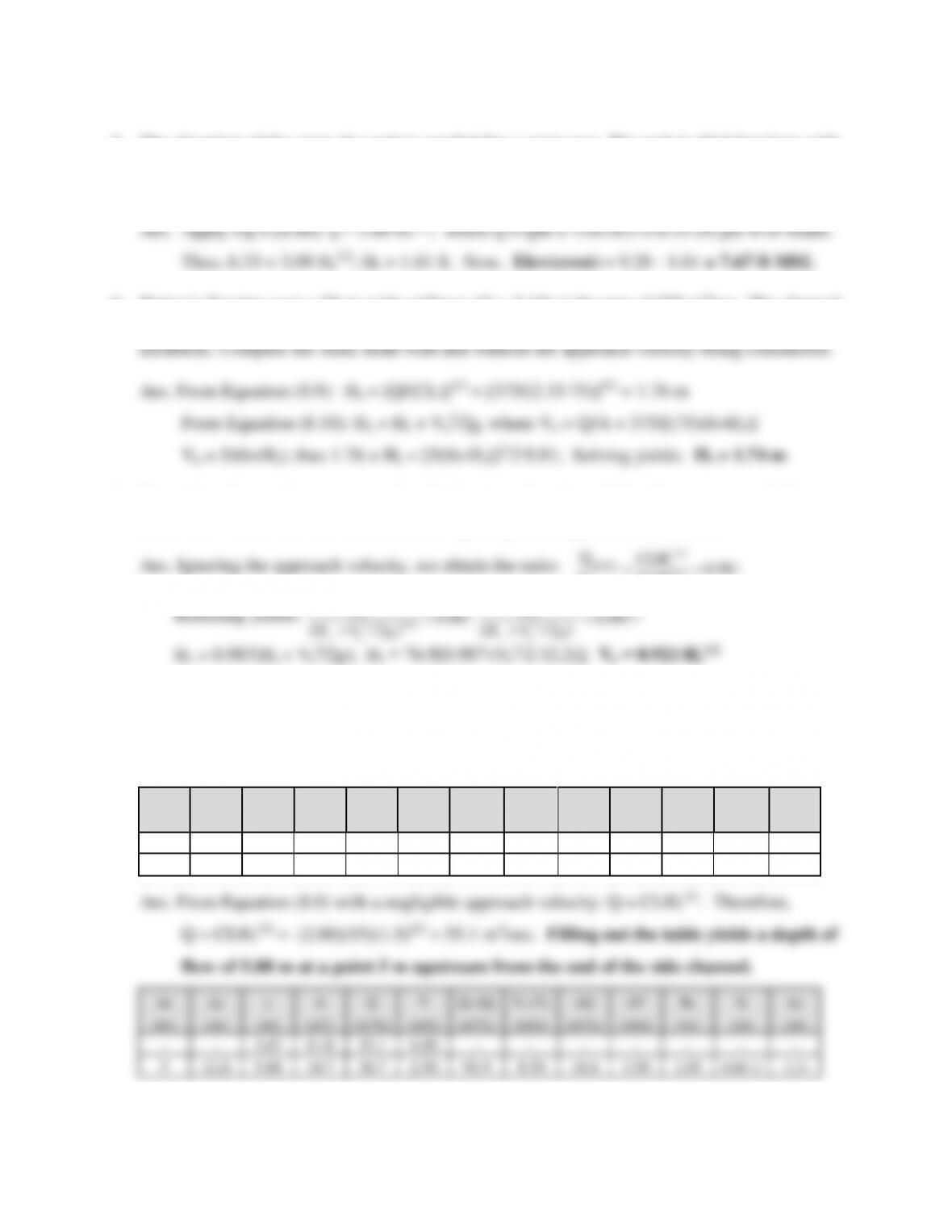85
5. The elevation of the crest of a weir is needed for a court case. The weir is 24.5 feet long with
a design flow of 155 cfs when the water surface elevation upstream is 9.28 feet above mean
sea level (MSL). Assume the weir friction loss and the velocity head upstream are negligible.
6. Water is flowing over a 75-m-wide spillway (C = 2.15) at the rate of 375 m3/sec. The channel
leading to the spillway is 6 meters deep at normal lake level (i.e., with no static head
7. Determine the maximum approach velocity (as a function of Hs) that can occur before an
error of 2% enters into the calculation for spillway discharge. (Use BG units.)
98.0
2/3
2/3
a
s
include
ignore
CLH
CLH
Q
Q
;
987.0
)2/( 2
gVH
H
as
s
;
8. An overflow spillway (C = 2.0), under a head of 1.5 m, discharges water into a side-channel
spillway that is 15 m long. At the end of the rectangular side channel, the depth of flow is
3.67 m. If the side channel has a bottom width of 2.5 meters (n = 0.015) and a bottom slope
of 1.00 %, determine the depth 5 meters upstream by filling in the table below.
Δx
Δy
y
A
Q
V
Q1+Q2
V1+V2
ΔQ
ΔV
Rh
Sf
Δy
(m)
(m)
(m)
(m2)
(m3/s)
(m/s)
(m3/s)
(m/s)
(m3/s)
(m/s)
(m)
(m)
(m)
-
-
3.67
5
-2.21
1.03
0.0013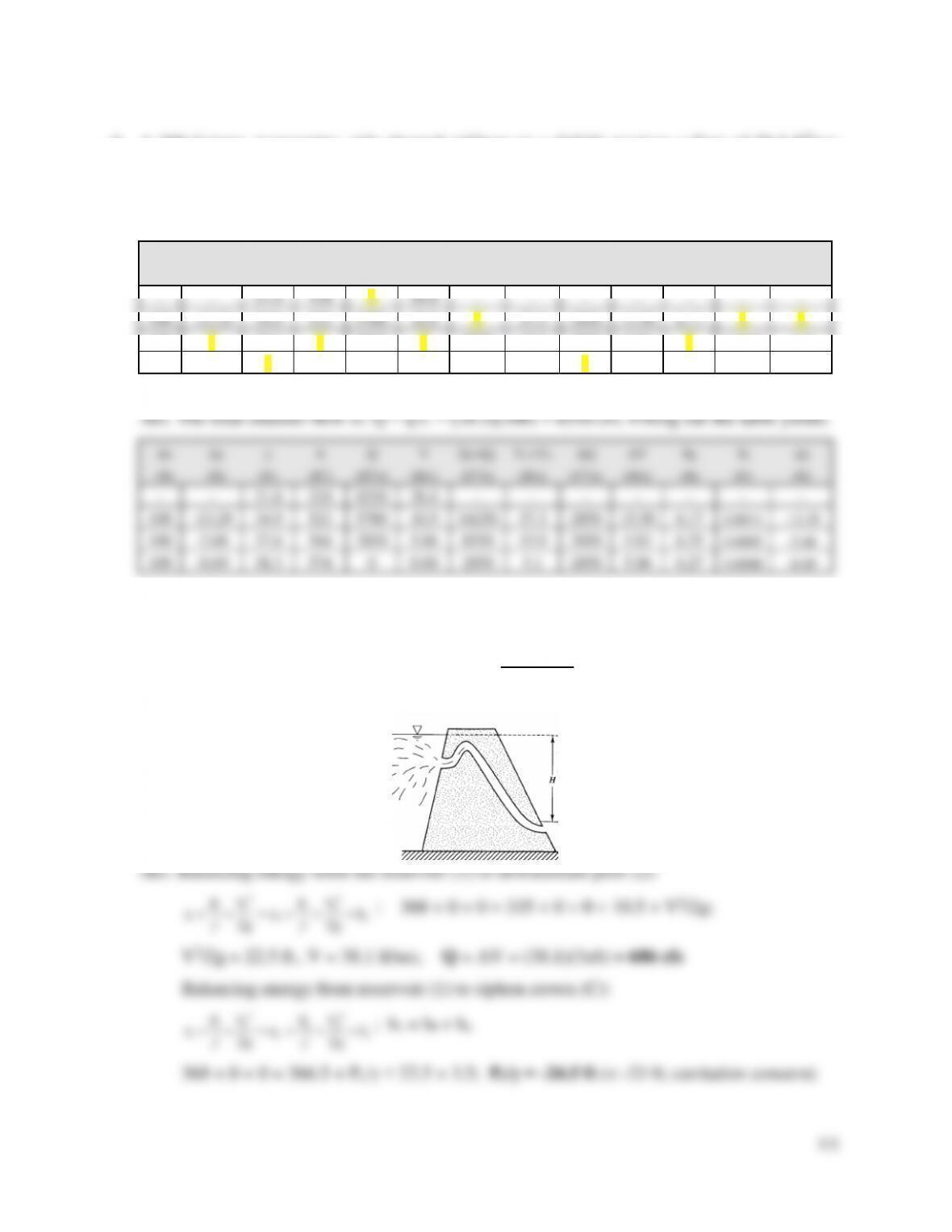86
9. A 300-ft-long, rectangular, side-channel spillway (n = 0.015) receives a flow of 28.5 ft3/sec
per foot of channel length from an overflow spillway (C = 3.73). The channel is 15 ft wide
and has a bottom slope of 0.001. The depth at the end of the channel is 21.6 feet. Define the
water surface profile (at 100-ft intervals) by filling in the blanks of the table below.
Δx
Δy
y
A
Q
V
Q1+Q2
V1+V2
ΔQ
ΔV
Rh
Sf
Δy
(ft)
(ft)
(ft)
(ft2)
(ft3/s)
(ft/s)
(ft3/s)
(ft/s)
(ft3/s)
(ft/s)
(ft)
(ft)
(ft)
-
21.6
324
26.4
-
-
-
-
-
-
-
100
34.9
523
5700
10.9
37.3
2850
15.50
6.17
?
?
100
?
37.6
?
2850
?
8550
15.9
2850
5.83
?
0.0002
-2.68
100
-0.69
?
574
0
0.00
2850
5.1
?
5.06
6.27
0.0000
-0.69
38.3
574
0
0.00
2850
5.1
2850
5.06
6.27
0.0000
10. A rectangular siphon (3 ft by 6 ft) discharges into a pool with an elevation of 335 ft, mean
sea level (MSL). Determine the discharge when the siphon primes at an upstream pool
elevation of 368 ft, MSL if losses are 10.5 ft excluding the exit loss. Also, determine the
pressure head at the siphon crown under these conditions if 3.5 ft of losses accrue prior to the
crown. The siphon crown is at an elevation of 366.5 ft.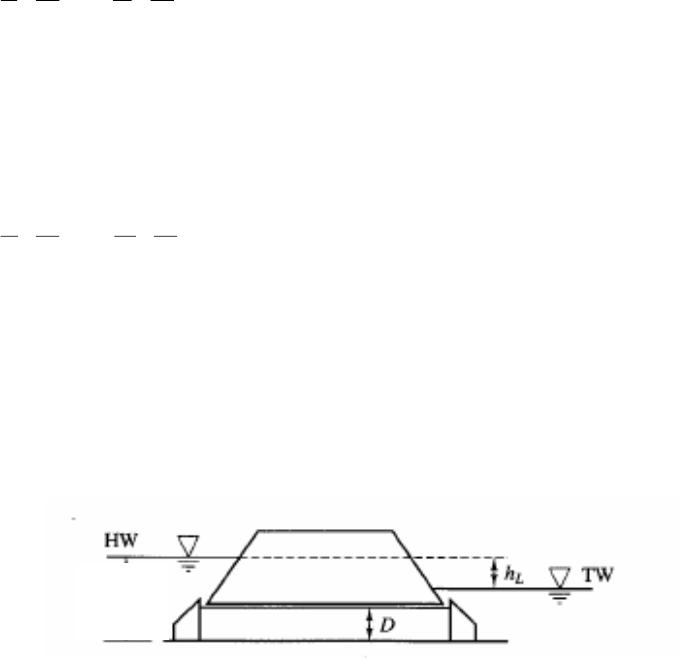87
11. A 60-m-long siphon spillway discharges water at the rate of 0.32 m3/sec. The siphon crown
is 1.2 m above the water surface elevation of the reservoir and a length of 10 m away from
the siphon entrance. If the siphon diameter is 30 cm, the friction factor is 0.02, and the head
loss coefficients are 0.2 (inlet) and 1.0 (exit), determine the elevation difference between the
siphon crown and the downstream pool. What is the pressure at the crown in kN/m2?
Ans. Balancing energy from reservoir to downstream pool:
L
h
g
VP
z
g
VP
z 22
2
22
2
2
11
1
; hL = hent + hf + hexit;
V = Q/A = (0.32)/[π(0.15)2] = 4.53 m/s
z1 + 0 + 0 = z2 + 0 + 0 + (0.2+0.02(60/0.3)+1.0)[4.532/2g]
z1 - z2 = 5.44 m; thus hc - h2 = 1.2 + 5.44 = 6.64 m (i.e., the elev. difference between
crown and downstream pool). Balancing energy from reservoir to siphon crown:
L
C
Ch
g
V
P
z
g
VP
z 22
2
2
11
1
; hL = hf + he
0 + 0 + 0 = 1.2 + Pc/γ + [4.532/2g] + (0.2+0.02(10/0.3))[4.532/2g];
Pc/γ = -3.15 m. Pc = (-3.15m)(9.79 kN/m3) = -30.8 kN/m2
12. Stage recorders register headwater and tailwater depths (4.05 m and 3.98 m) of a culvert
during a flood event. The 2 m by 2 m concrete culvert (square-edged entrance) is 15 m long
and has a slope of 3.0%. Based on this information, determine the flood flow rate that passed
through the culvert. The stream depths are measured above the culvert inverts (bottom).
Ans. Since the outlet is submerged, the hydraulic operation category is (a) and the energy
balance is: Hup + SoL = Hdown + hL; where H = stream depths
4.05 + (0.03)(15) = 3.98 + hL; hL = 0.52 m
The head loss from hydraulic category (a) is:
hL = [Ke + {n2L/Rh4/3}(2g) + 1]{Q2/(2g∙A2)};
where Rh = A/P = (4 m2)/(8 m) = 0.5 m. Thus,
0.52 = [0.5+{0.0132(15)/(0.5)4/3}(2g)+1]{Q2/(2g(42)}; Q = 10.0 m3/sec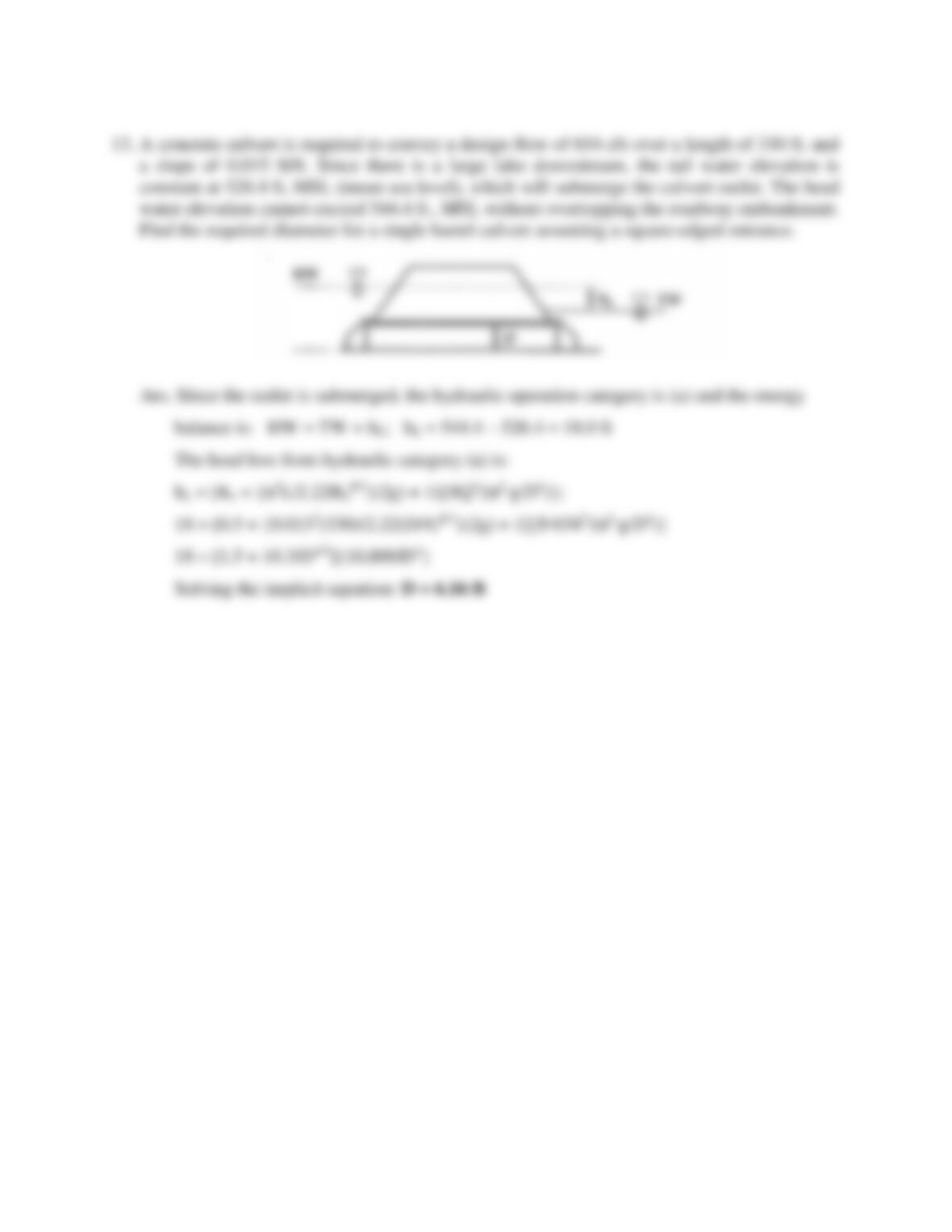88
13. A concrete culvert is required to convey a design flow of 654 cfs over a length of 330 ft. and
a slope of 0.015 ft/ft. Since there is a large lake downstream, the tail water elevation is
constant at 526.4 ft, MSL (mean sea level), which will submerge the culvert outlet. The head
water elevation cannot exceed 544.4 ft., MSL without overtopping the roadway embankment.
Find the required diameter for a single barrel culvert assuming a square-edged entrance.
Ans. Since the outlet is submerged, the hydraulic operation category is (a) and the energy
balance is: HW = TW + hL; hL = 544.4 526.4 = 18.0 ft
The head loss from hydraulic category (a) is:
hL = [Ke + {n2L/2.22Rh4/3}(2g) + 1]{8Q2/(π2∙g∙D4)};
18 = [0.5 + {0.0132(330)/2.22(D/4)4/3}(2g) + 1]{8∙6542/(π2∙g∙D4)}
18 = [1.5 + 10.3/D4/3]{10,800/D4}
Solving the implicit equation: D = 6.16 ft

## Trusted by Thousands ofStudents

Here are what students say about us.